# Q6. Let W be the subspace of R' spanned by the vectors u. = 3(1, -1,1,1),...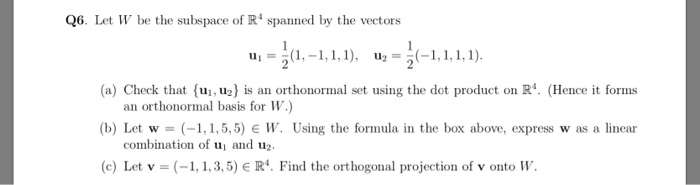Q6. Let W be the subspace of R' spanned by the vectors u. = 3(1, -1,1,1), uz = 5(–1,1,1,1). (a) Check that {uj,uz) is an orthonormal set using the dot product on R. (Hence it forms an orthonormal basis for W.) (b) Let w = (-1,1,5,5) EW. Using the formula in the box above, express was a linear combination of u and u. (c) Let v = (-1,1,3,5) = R'. Find the orthogonal projection of v onto W.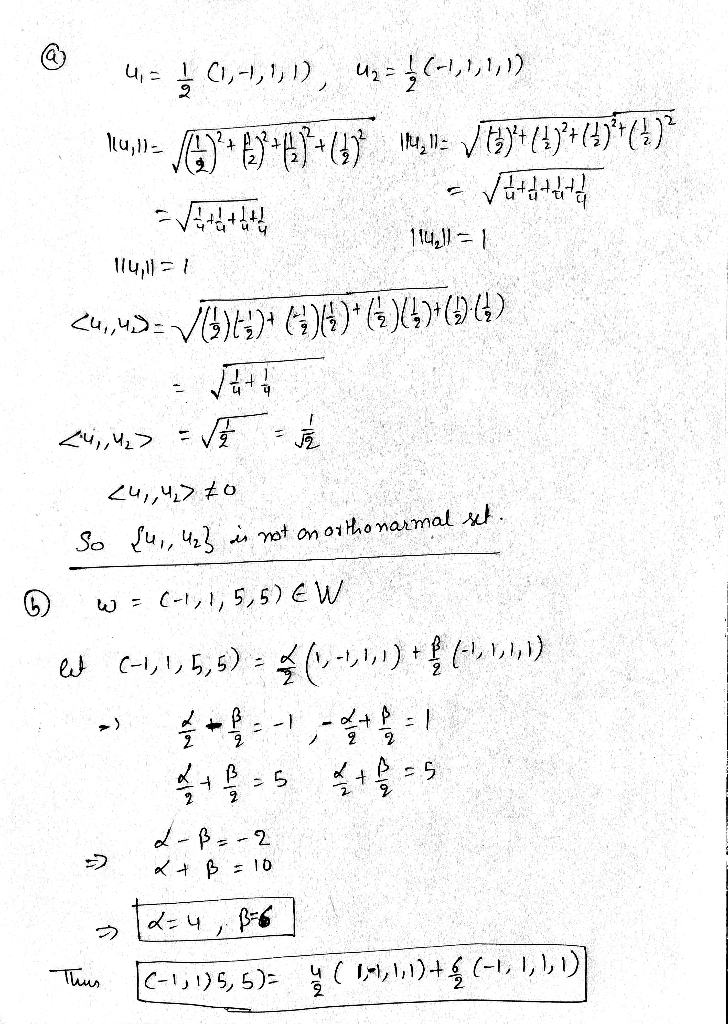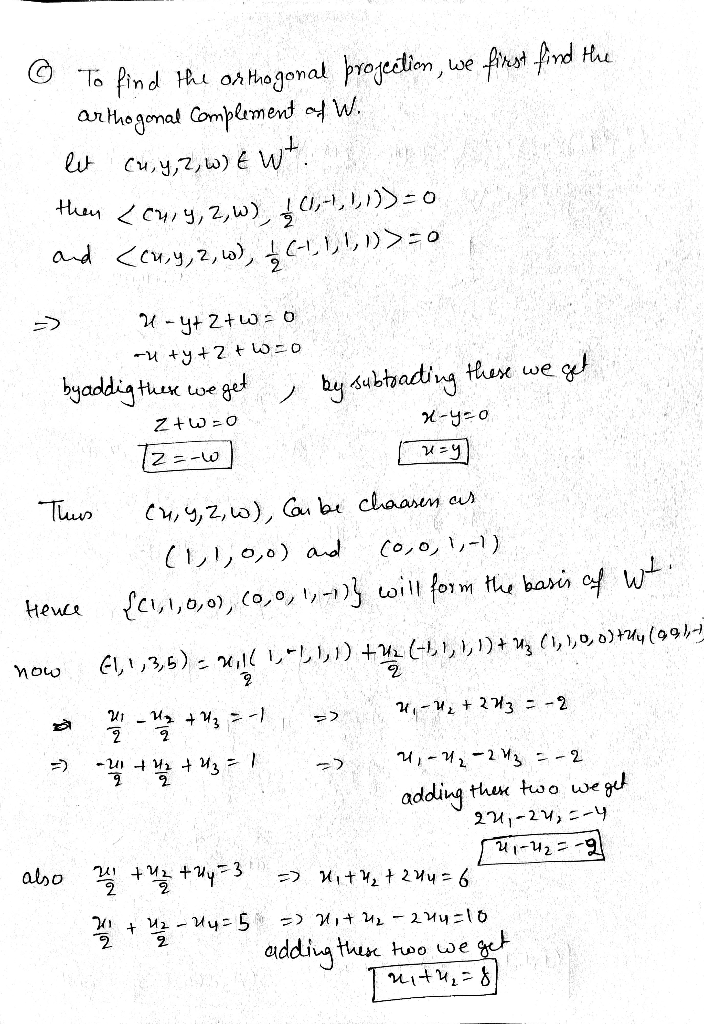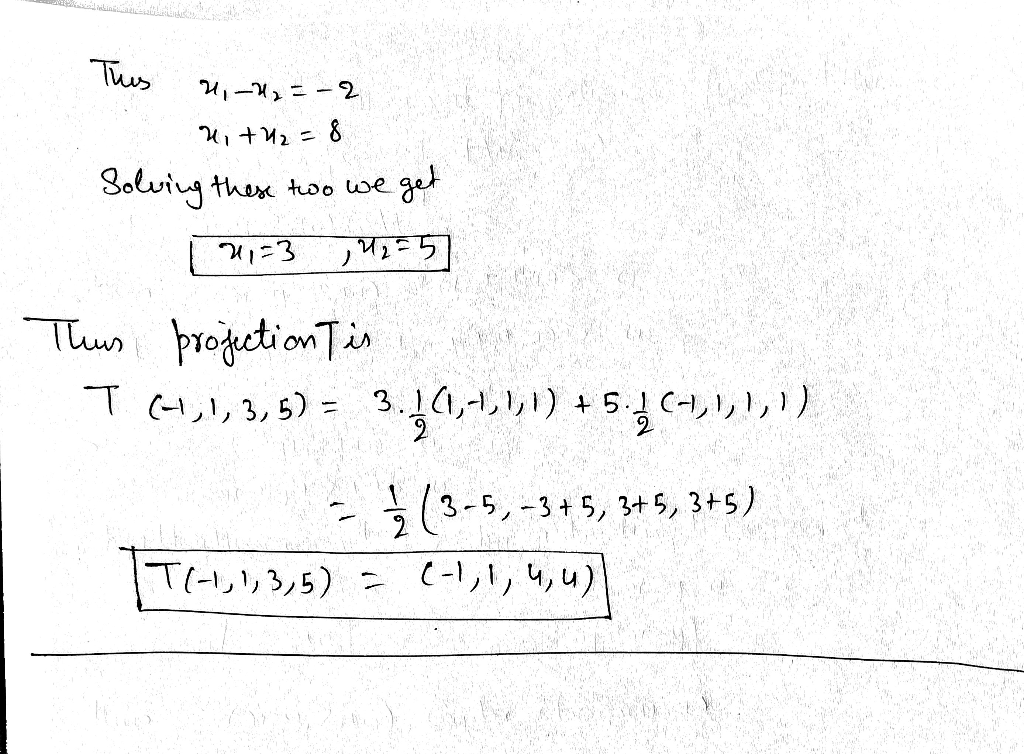#### Earn Coin

Coins can be redeemed for fabulous gifts.

Similar Homework Help Questions
• ### (3 points) Let W be the subspace of R spanned by the vectors 1and 5 Find the matrix A of the orthogonal projection onto W A- (3 points) Let W be the subspace of R spanned by the vectors 1and 5 F...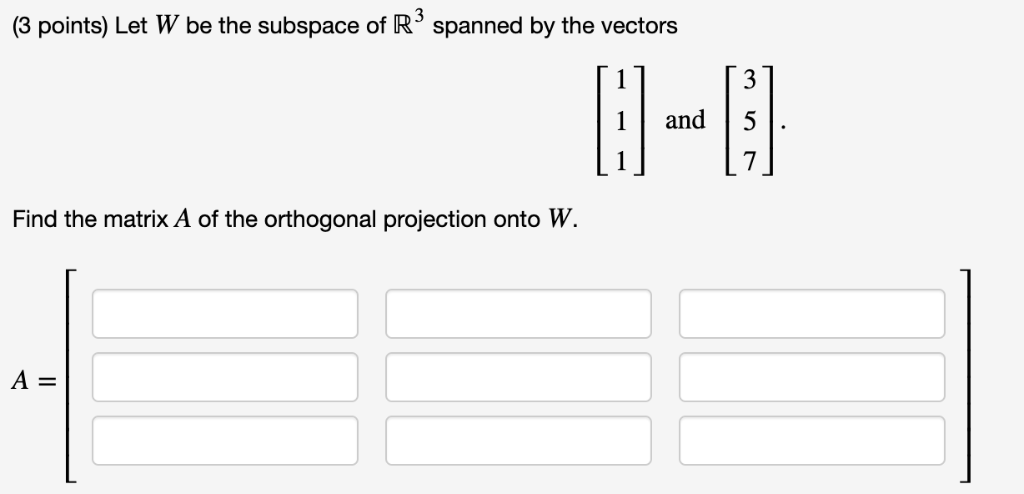(3 points) Let W be the subspace of R spanned by the vectors 1and 5 Find the matrix A of the orthogonal projection onto W A- (3 points) Let W be the subspace of R spanned by the vectors 1and 5 Find the matrix A of the orthogonal projection onto W A-

• ### (1 point) Let W be the subspace of R spanned by the vectors 27 1 and...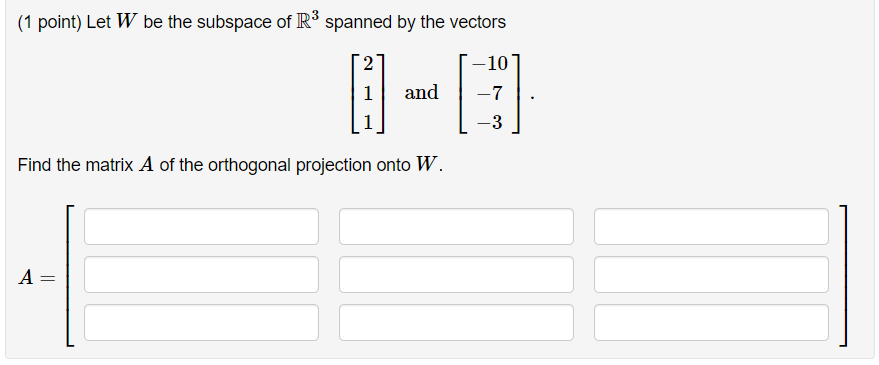(1 point) Let W be the subspace of R spanned by the vectors 27 1 and -7 Find the matrix A of the orthogonal projection onto W. A =

• ### (12 points) Let vi = 1 and let W be the subspace of R* spanned by...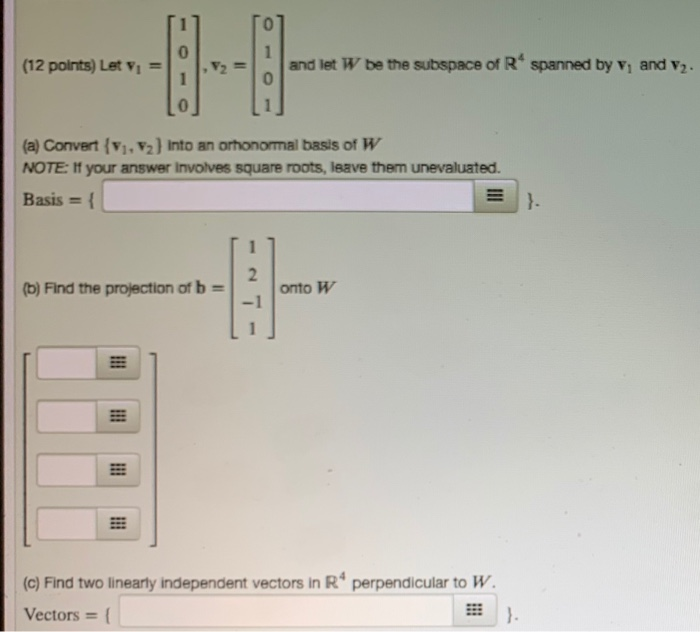(12 points) Let vi = 1 and let W be the subspace of R* spanned by V, and v. (a) Convert (V. 2) into an ohonormal basis of W NOTE: If your answer involves square roots, leave them unevaluated. Basis = { (b) Find the projection of b = onto W (c) Find two linearly independent vectors in R* perpendicular to W. Vectors = 1

• ### All vectors and subspaces are in R”. Mark each statement True or False. Justify each answer....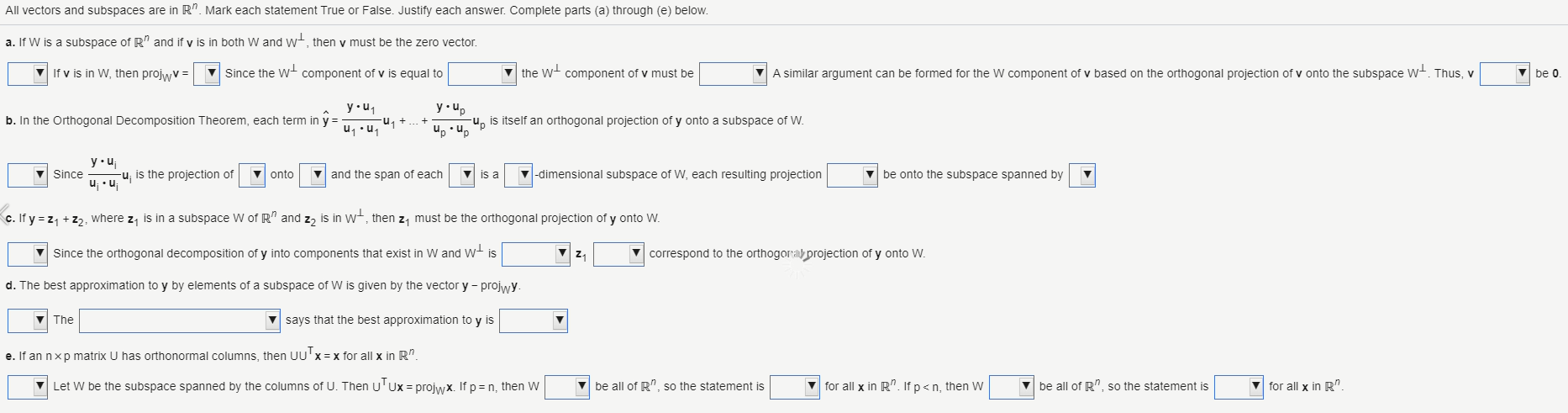All vectors and subspaces are in R”. Mark each statement True or False. Justify each answer. Complete parts (a) through (e) below. a. If W is a subspace of R" and if y is in both W and wt, then y must be the zero vector. If v is in W, then projwv = Since the wt component of v is equal to v the w+ component of v must be A similar argument can be formed for the W...

• ### 11 -14 (1 point) Let W be the subspace of R3 spanned by the vectors 1...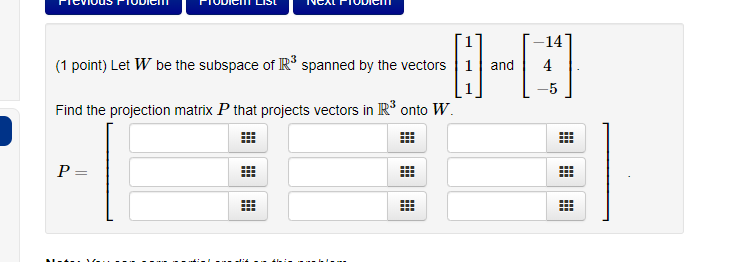11 -14 (1 point) Let W be the subspace of R3 spanned by the vectors 1 and 4 Find the projection matrix P that projects vectors in R3 onto W

• ### e, none of these 7. Let {1,..., up} be an orthogonal basis for a subspace W...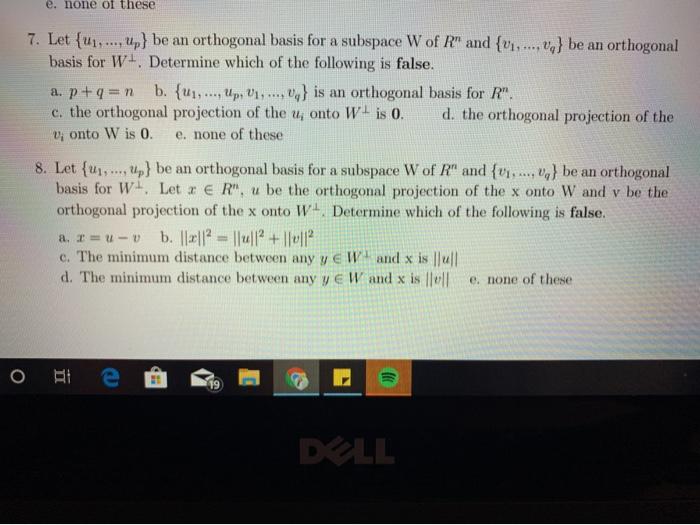e, none of these 7. Let {1,..., up} be an orthogonal basis for a subspace W of R" and {...., } be an orthogonal basis for Wt. Determine which of the following is false. a. p+q=n b. {U1,..., Up, V1,...,0} is an orthogonal basis for R". c. the orthogonal projection of the u; onto W is 0. d. the orthogonal projection of the vi onto W is 0. e. none of these 8. Let {u},..., up} be an orthogonal basis...

• ### Let w be a subspace of R", and let wt be the set of all vectors...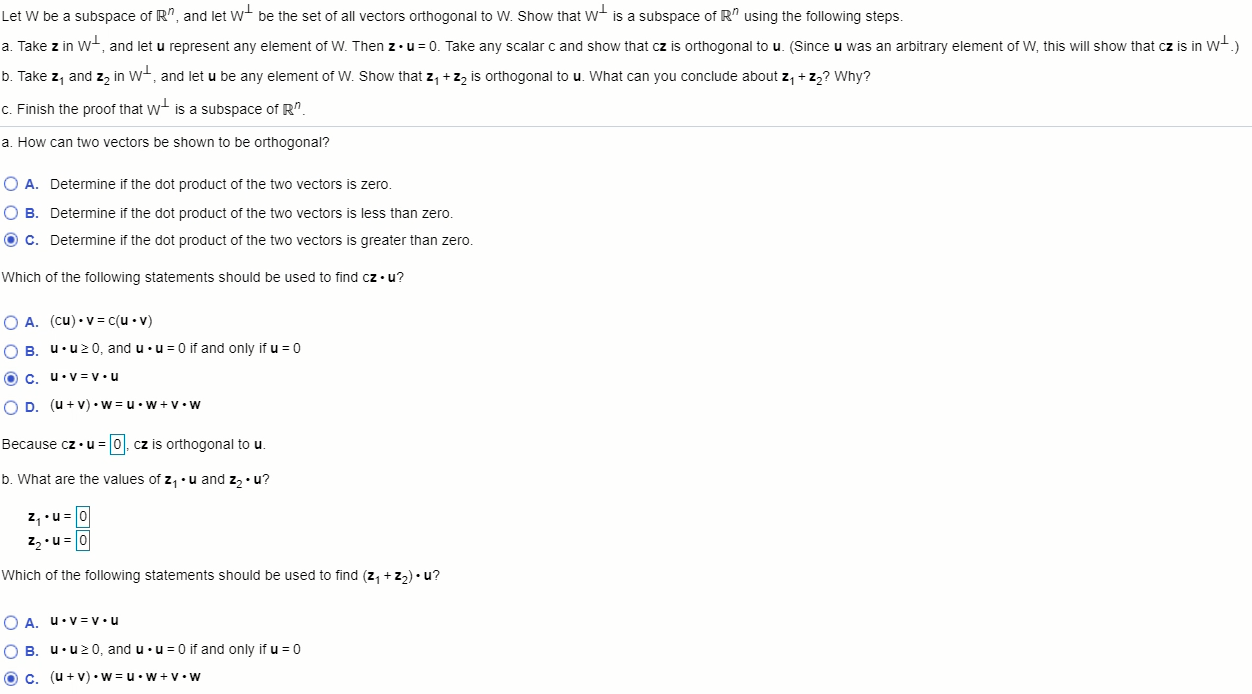Let w be a subspace of R", and let wt be the set of all vectors orthogonal to W. Show that wt is a subspace of R" using the following steps. a. Take z in wt, and let u represent any element of W. Then zu u = 0. Take any scalar c and show that cz is orthogonal to u. (Since u was an arbitrary element of W, this will show that cz is in wt.) b. Take z,...

• ### -9 2. Let Vi-8.V2,andvs-2, let B -(V,V2,Vs), and let W be the subspace spanned , let B -(Vi,V2,V3...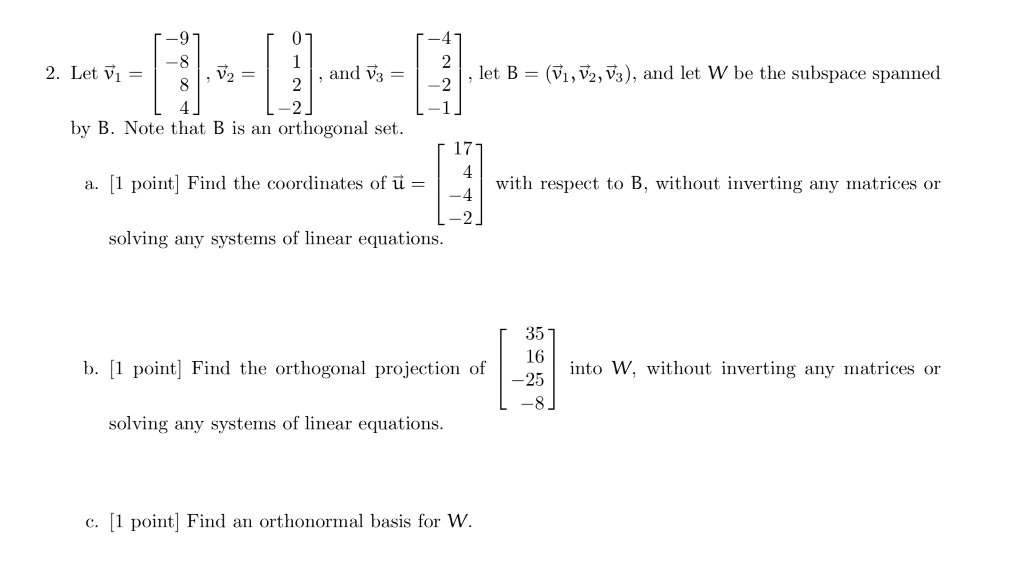-9 2. Let Vi-8.V2,andvs-2, let B -(V,V2,Vs), and let W be the subspace spanned , let B -(Vi,V2,V3), and let W be the subspace spanned by B. Note that B is an orthogonal set. 17 a. 1 point] Find the coordinates of uwith respect to B, without inverting any matrices or L-2 solving any systems of linear equations. 35 16 25 b. 1 point Find the orthogonal projection of to W, without inverting any matrices or solving any systems of...

• ### Let W be the subspace of R3 spanned by the vectors ⎡⎣⎢113⎤⎦⎥ and ⎡⎣⎢4615⎤⎦⎥. Find the projection matrix P that project...

Let W be the subspace of R3 spanned by the vectors ⎡⎣⎢113⎤⎦⎥ and ⎡⎣⎢4615⎤⎦⎥. Find the projection matrix P that projects vectors in R3 onto W.

• ### 0/1 pts Inooreat Question 9 Suppose W is a subspace of R" spanned by n nonzero...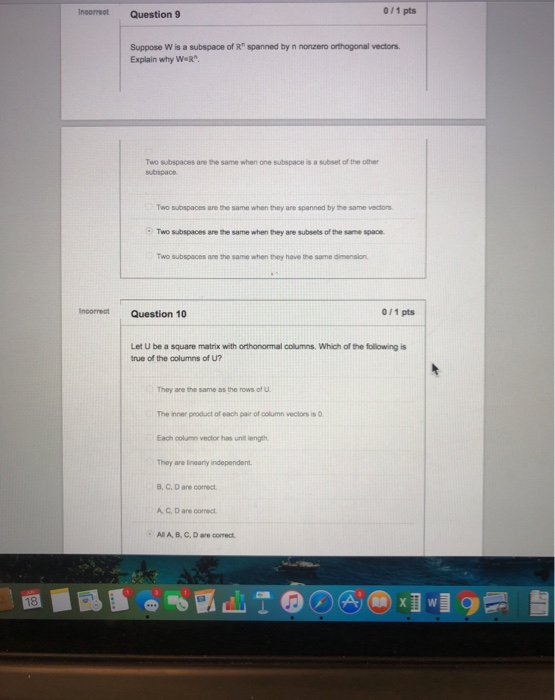0/1 pts Inooreat Question 9 Suppose W is a subspace of R" spanned by n nonzero orthogonal vectors. Explain why WR Two subspaces are the same when one subspace is a subset of the other subspace. Two subspaces are the same when they are spanned by the same vectors Two subspaces are the same when they are subsets of the same space Two subspaces are the same when they have the same dimension Incorrect 0/1 pts Question 10 Let U...